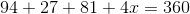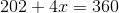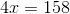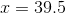# ISEE Upper Level Math : How to find an angle in other quadrilaterals

## Example Questions

### Example Question #1 : Other Quadrilaterals

Three of the interior angles of a quadrilateral measure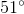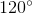, and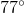. What is the measure of the fourth interior angle?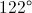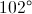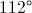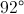Explanation:

The measures of the angles of a quadrilateral have sum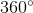. Ifis the measure of the unknown angle, then: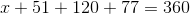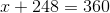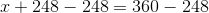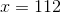The angle measures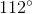.

### Example Question #2 : Other Quadrilaterals

The angles of a quadrilateral measure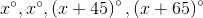. Evaluate.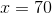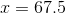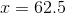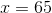Explanation:

The sum of the degree measures of the angles of a quadrilateral is 360, so we can set up and solve forin the equation: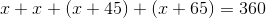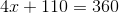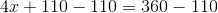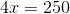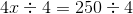### Example Question #3 : Other Quadrilaterals

The four angles of a quadrilateral have the following value: 79 degrees, 100 degrees, 50 degrees, anddegrees. What is the value of?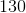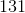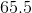Explanation:

Given that there are 360 degrees when all the angles of a quadrilateral are added toghether, this problem can be solved with the following equation: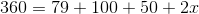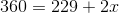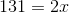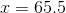### Example Question #4 : Other Quadrilaterals

In a quadrilateral, the angles have the following values: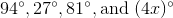What is the value of?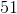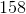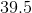Explanation:

Given that there are 360 degrees when the angles of a quadrilateral are added together, it follows that: